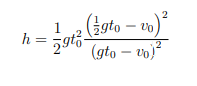# Hi, new here, im really stuggling on how to even start this question any help would be much appreicated!

### Hi, new here, im really stuggling on how to even start this question any help would be much appreicated!​

A ball is thrown straight up from the edge of the roof of a building of height h, with an initial speed of v0. A second ball is dropped from the roof t0 later. Ignore air resistance. 2 (a) If the balls reach the ground at the same time, show that the height is

Thanks in advance!#### mmm4444bot

##### Super Moderator
Staff member
Hello. Please share what you've already learned about exercises involving displacements, velocities and times. What have you thought about so far? Have you drawn a diagram?

#### HallsofIvy

##### Elite Member
What DO you know about this problem? Do you know about gravity? That there is a downward force on every object proportional to its mass so that there is a constant downward acceleration of approximately g= 9.8 meters per second per second (or g= 32.2 feet per second per second).

The first ball is thrown up (from height h) with initial velocity $$\displaystyle v_0$$ so, with acceleration g, after t seconds its speed will be $$\displaystyle v_0- gt$$ and it will have reached height $$\displaystyle h+ v_0t- (g/2)t^2$$. It will be 0 (the ball willl have hit the ground) when $$\displaystyle h+ v_0t- (g/2)t^2= 0$$. That is a quadratic equation that has two roots, one positive and one negative. Since the ball cannot hit the ground until after it is thrown, solve for the positive root.

At time $$\displaystyle t_0$$, a second ball is dropped from height h. It also has downward acceleration g but has 0 initiall speed so after time t has downard speed -gt and will be a height h- gt. That ball hits the ground when $$\displaystyle h- g(t-t_0)= 0$$ or $$\displaystyle t= t_0+ h/g$$.

"If the two balls hit the ground at the same time" set those times equal and solve for h.

Last edited:
•Jomo and Subhotosh Khan